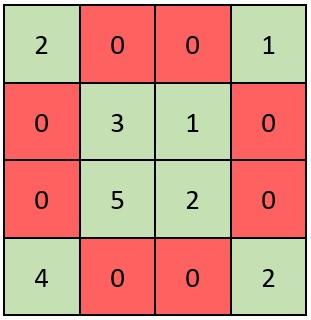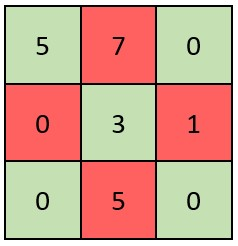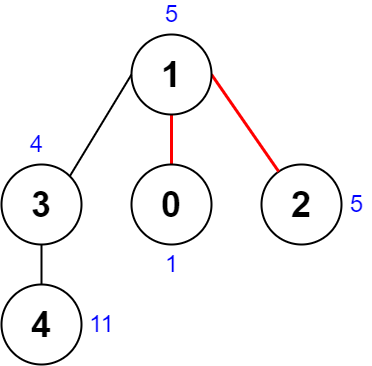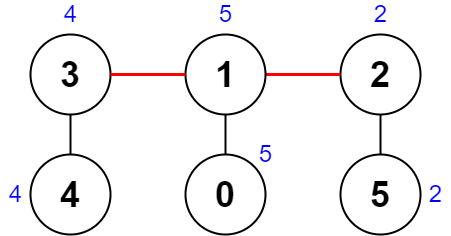# 战局详情

639 / 6108Juruoer120:36:290:04:270:15:560:31:29 1

# 题目及解答

2319. 判断矩阵是否是一个 X 矩阵

• 矩阵对角线上的所有元素都 不是 0
• 矩阵中所有其他元素都是 0

• n == grid.length == grid[i].length
• 3 <= n <= 100
• 0 <= grid[i][j] <= 1e5X 矩阵应该满足：绿色元素（对角线上）都不是 0 ，红色元素都是 0 。X 矩阵应该满足：绿色元素（对角线上）都不是 0 ，红色元素都是 0 。

2320. 统计放置房子的方式数

• 1 <= n <= 1e4

1. 所有地块都不放置房子。
2. 一所房子放在街道的某一侧。
3. 一所房子放在街道的另一侧。
4. 放置两所房子，街道两侧各放置一所。• dp[i] 表示自第 0 个地块起到第 i 个地块为止，且第 i 个地块 不摆放 房子的方式数，它是前一个位置摆放房子的方式数和前一个位置不摆放房子的方式数的总和。
• dp[i] 表示自第 0 个地块起到第 i 个地块为止，且第 i 个地块 摆放 房子的方式数，它与前一个位置不摆放房子的方式数相同（两所房子不能相邻）。

• dp[i] = dp[i - 1] + dp[i - 1]
• dp[i] = dp[i - 1]

2321. 拼接数组的最大分数

• 例如，设 nums1 = [1,2,3,4,5]nums2 = [11,12,13,14,15] ，整数选择 left = 1right = 2，那么 nums1 会变为 [1,12,13,4,5]nums2 会变为 [11,2,3,14,15]

• n == nums1.length == nums2.length
• 1 <= n <= 1e5
• 1 <= nums1[i], nums2[i] <= 1e4

• 最终结果为 nums2 的子数组被交换到了 nums1 中。
• 最终结果为 nums1 的子数组被交换到了 nums2 中。

dp[j] 表示 ii 在什么位置无需知道）到 j 范围内交换元素，nums1 可以获得的增量，任何情况下 dp[j] >= 0 ，当增量为负时，范围起点变为 j 增量变为 0 相当于不交换元素的增量。在此过程中，用 _max 记录最大的增量。则有：

• dp[j] = max(dp[j - 1] + (nums2[j] - nums1[j]), 0);
• _max = _max(_max, dp[j]);

2322. 从树中删除边的最小分数

1. 分别获取三个组件 每个 组件中所有节点值的异或值。
2. 最大 异或值和 最小Z 异或值的 差值 就是这一种删除边方案的分数。
• 例如，三个组件的节点值分别是：[4,5,7][1,9][3,3,3] 。三个异或值分别是 4 ^ 5 ^ 7 = 61 ^ 9 = 83 ^ 3 ^ 3 = 3 。最大异或值是 8 ，最小异或值是 3 ，分数是 8 - 3 = 5
返回在给定树上执行任意删除边方案可能的 最小 分数。

• n == nums.length
• 3 <= n <= 1000
• 1 <= nums[i] <= 1e8
• edges.length == n - 1
• edges[i].length == 2
• 0 <= ai, bi < n
• ai != bi
• edges 表示一棵有效的树• 第 1 个组件的节点是 [1,3,4] ，值是 [5,4,11] 。异或值是 5 ^ 4 ^ 11 = 10 。
• 第 2 个组件的节点是  ，值是  。异或值是 1 = 1 。
• 第 3 个组件的节点是  ，值是  。异或值是 5 = 5 。• 第 1 个组件的节点是 [3,4] ，值是 [4,4] 。异或值是 4 ^ 4 = 0 。
• 第 2 个组件的节点是 [1,0] ，值是 [5,5] 。异或值是 5 ^ 5 = 0 。
• 第 3 个组件的节点是 [2,5] ，值是 [2,2] 。异或值是 2 ^ 2 = 0 。

DFS：

• tree[y1]tree[y2] 的子树：x = _xor[y1], y = _xor[y2] ^ x, z = _xor ^ _xor[y2] 。
• tree[y2]tree[y1] 的子树：x = _xor[y2], y = _xor[y1] ^ x, z = _xor ^ _xor[y1] 。
• tree[y2]tree[y1] 没有交集：x = _xor[y1], y = _xor[y2], z = _xor ^ x ^ y;

• in[i] < in[j] < out[j] < out[i]，那么表明对 j 的递归是在对 i 的递归的过程中完成的，也就是说，ji 的子孙节点，由递归的过程可知，当满足 in[i] < in[j] < out[i] 时一定满足 out[j] < out[i]